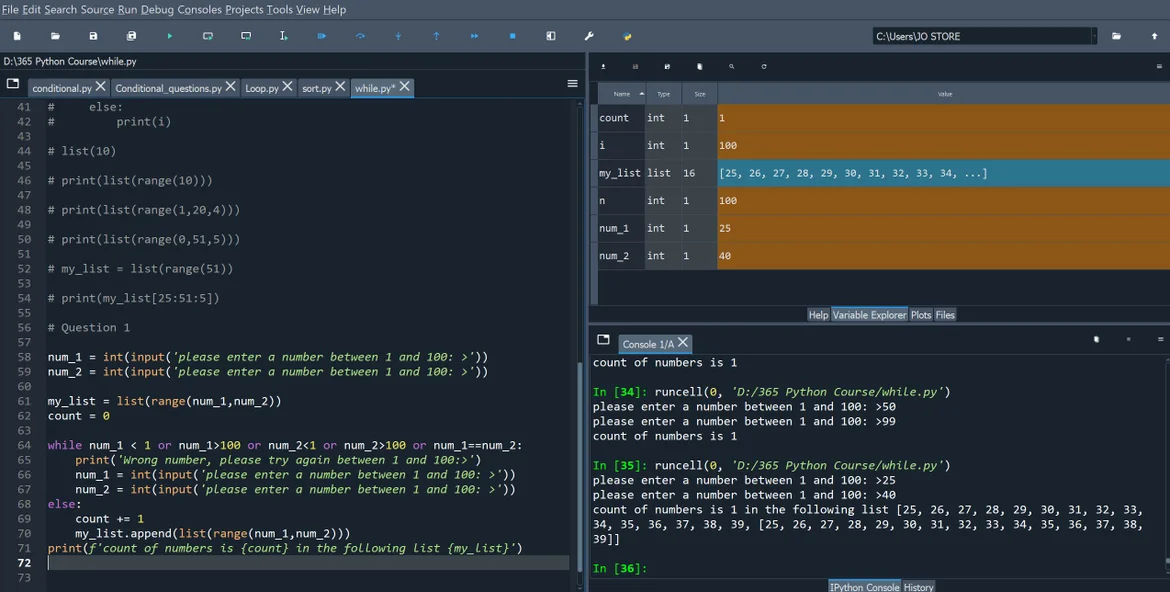19 Jul 2023

Posted on:

18 Jul 2023

0

# Resolved:Question 1 answer - count

Hi Hristina,

Have a good day.

Regarding question 1 , you asked to count from the lowest to the highest number, so i understood that you needed the count as the answer, so i tried the following code, but it kept giving me a count of 0 or 1, so what could be wrong ?Instructor
Posted on:

19 Jul 2023

0

Hey Mohamed,

Let's discuss the `while`-loop. Note that the `else` keyword is only used in an `if-else` construction. Therefore, the first modification I would suggest is removing the `else` keyword and unindenting the code in lines 69 and 70, so that they stay outside of the loop:

``````while num_1 < 1 or num_1 > 100 or num_2 < 1 or num_2 > 100 or num_1 == num_2:
print('try again')
num_1 = int(input('please enter a number between 1 and 100: '))
num_2 = int(input('please enter a number between 1 and 100: '))

count += 1
my_list.append(list(range(num_1, num_2)))``````

Next, note that

``count += 1``

is executed only once—when the conditions for the `num_1` and `num_2`  variables are satisfied and you exit the `while`-loop. If you want to get the count of the numbers, I would suggest either finding the length of the list that stores the numbers (`my_list` in your case), or computing it as follows:

``count = num_2 - num_1 + 1``

Which brirngs me to my comment regarding the `my_list` variable. Notice that in line 61, you create a list of the numbers between `num_1` and `num_2`. However, they might be numbers outside the range [1, 100]. I would therefore suggest removing the code in line 70 and moving the code from line 61 to line 70, i.e.

``````num_1 = int(input('please enter a number between 1 and 100: '))
num_2 = int(input('please enter a number between 1 and 100: '))

while num_1 < 1 or num_1 > 100 or num_2 < 1 or num_2 > 100 or num_1 == num_2:
print('try again')
num_1 = int(input('please enter a number between 1 and 100: '))
num_2 = int(input('please enter a number between 1 and 100: '))

count = num_2 - num_1 + 1
my_list = list(range(num_1, num_2))

print(f'count: {count} \nlist: {my_list}')``````

Next, note that the `range()` function that you use to create `my_list` includes `num_1` but excludes `num_2`. Therefore, in order to include `num_2` to the list of numbers, you would have to add `+1` like so:

``my_list = list(range(num_1, num_2+1))``
Instructor
Posted on:

19 Jul 2023

0

And finally, my last comment thta I would leave to you to resolve is that your code assumes that `num_1` is smaller than `num_2`. That, however, doesn't have to be the case. Think about how you can modify your code, so that it creates the list of numbers in both cases:

1. `num_1 `< `num_2`

2. `num_1 `> `num_2`

Hope this helps! Let me know if you experience other issues.

Kind regards,

365 Hristina

Posted on:

19 Jul 2023

0

Thank you so much for your help.

I also solved the last issue that you mentioned by if condition code in Bold, so my final code is:

num_1 = int(input('please enter a number between 1 and 100: >'))
num_2 = int(input('please enter a number between 1 and 100: >'))

while num_1 < 1 or num_1>100 or num_2<1 or num_2>100 or num_1==num_2:
print('Wrong number, please try again between 1 and 100:>')
num_1 = int(input('please enter a number between 1 and 100: >'))
num_2 = int(input('please enter a number between 1 and 100: >'))

if num_1>num_2:
num_1,num_2 = num_2,num_1

count = len(range(num_1,num_2+1))
my_list = list(range(num_1,num_2+1))

print(f'count of numbers is {count} in the following list {my_list}')

Instructor
Posted on:

19 Jul 2023

1

Hey again Mohamed,

Excellent solution!

Kind regards,

365 Hristina